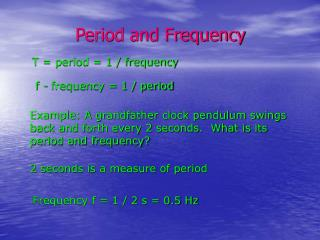DownloadDownload PresentationT = period = 1 / frequency

# T = period = 1 / frequency

Télécharger la présentation## T = period = 1 / frequency

- - - - - - - - - - - - - - - - - - - - - - - - - - - E N D - - - - - - - - - - - - - - - - - - - - - - - - - - -
##### Presentation Transcript

1. Period and Frequency T = period = 1 / frequency f - frequency = 1 / period Example: A grandfather clock pendulum swings back and forth every 2 seconds. What is its period and frequency? 2 seconds is a measure of period Frequency f = 1 / 2 s = 0.5 Hz

2. Types of Waves Mechanical need a physical medium for travel sound, surf, shock waves Electromagnetic can travel through a vacuum light, radio, TV, microwaves

3. Transverse Waves When the motion of the particles of the medium is perpendicular to the motion of the wave. The wave has crests, troughs, amplitude and wavelength.

4. Longitudinal Waves When the motion of the particles of the medium is parallel to the motion of the wave. The wave has compressions, rarefactions and wavelengths.

5. Measurements Of Waves λ = Wavelength = distance between two successive points in phase v = velocity (speed) of a wave

6. Measurements Of Waves A = Amplitude of a wave The maximum displacement from the equilibrium position

7. Amplitude of a Wave The maximum displacement from the equilibrium position – proportional to the energy in the wave. Can be the intensity of an earthquake, the loudness of a sound wave or the energy in a tsunami.

8. Measurement of waves Wavelength (λ) – distance between two successive points in phase Frequency (f) – the number of waves passing a point each second Velocity (v) – speed the wave is traveling through the medium v = f xλor v = λ / T

9. Sample Problem #1 A sound wave travels at 340 m/s. If the frequency of the sound it 256 Hz, what is the wavelength? v = f λ or 340 m/s = 256 Hz λ λ = 340 m/s / 256 Hz = 1.33 m

10. Sample Problem #2 A sound wave travels at 340 m/s. If the frequency of the sound it 256 Hz, what is the wavelength? v = f λ or 340 m/s = 256 Hz λ λ = 340 m/s / 256 Hz = 1.33 m